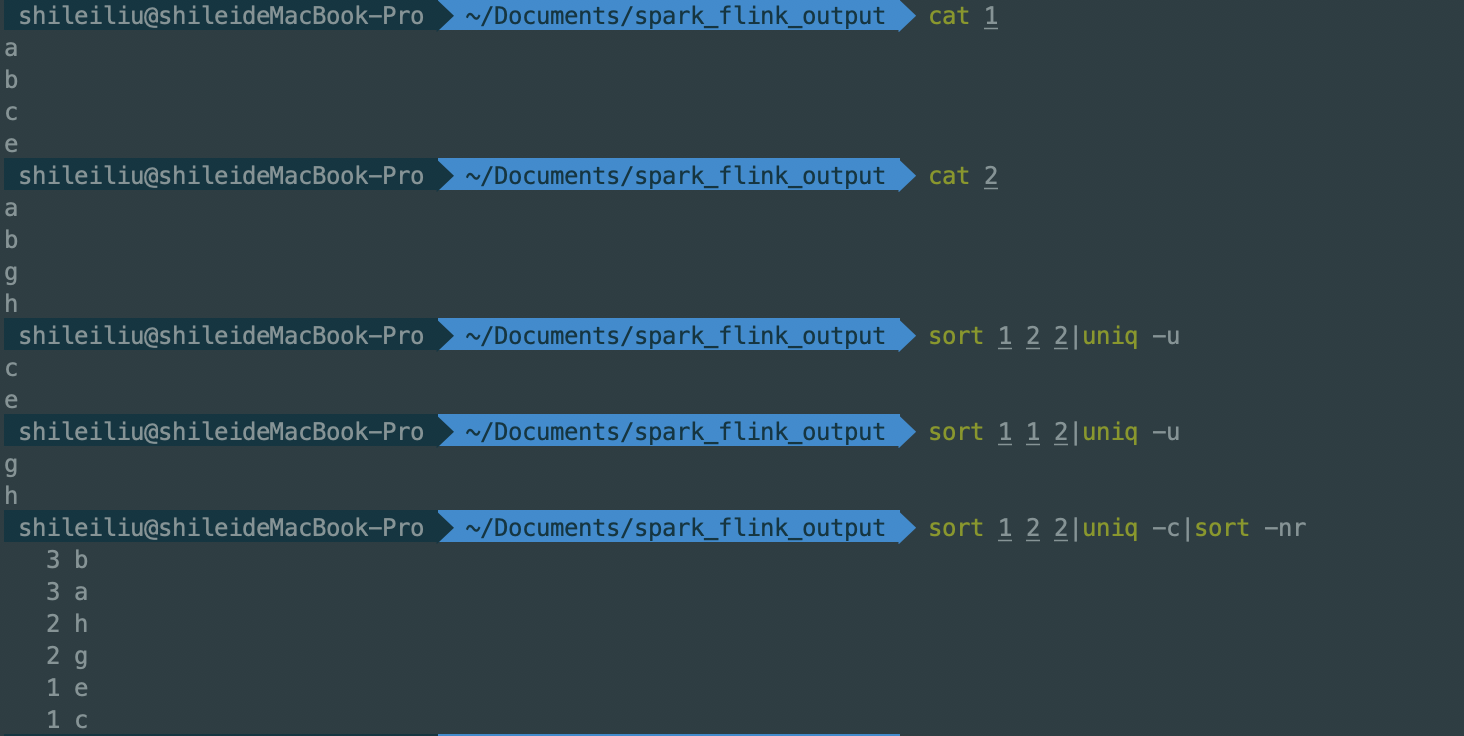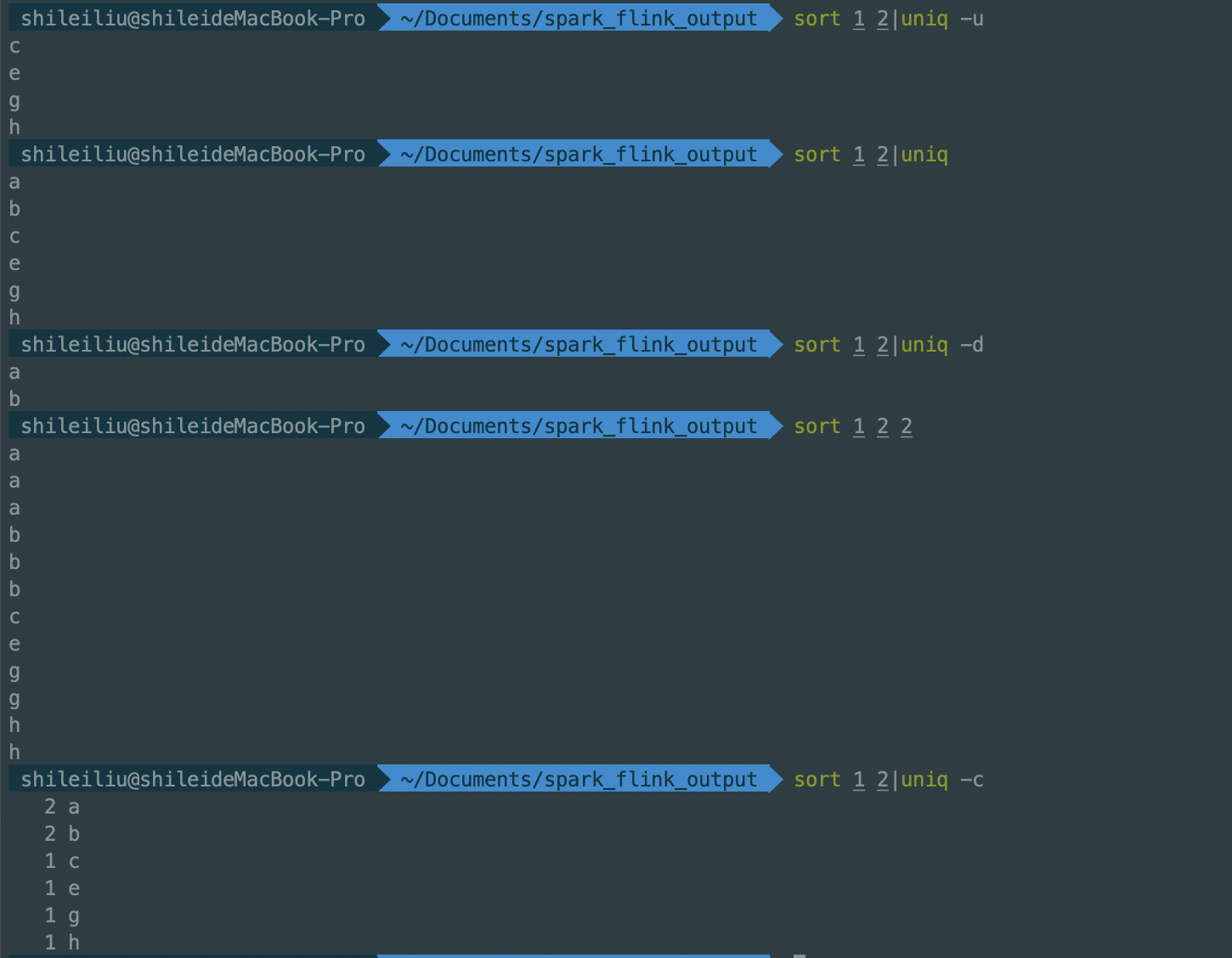• uniq -u 取出只出现过一次的元素，用来求差集 uniq -c group by ...所以求交集并集差集的关键不是在sort, 而是在uniq后面的选项 如果单纯只有uniq, 就是单纯的去重，其实就是取并集 所以sort a b|un...
uniq -u    取出只出现过一次的元素，用来求差集

uniq -c     group by

uniq -d     取出出现次数大于等于2的元素，用来求交集

sort 1 2 和cat 1 2 本质是一样的，只不过sort排序了

所以求交集并集差集的关键不是在sort, 而是在uniq后面的选项

如果单纯只有uniq, 就是单纯的去重，其实就是取并集

所以sort a b|uniq 这个命令表示的含义有

1.对两个文件排序并去重，等同cat a b|sort -u

特别注意想对文件整体去重一定要先排序，uniq只能对相邻重复元素去重

2.取两个文件的并集想起之前遇到过的几个面试题

1.在一份日志中统计出所有包含error的行中出现的ip, 统计他们出现的次数，并倒序排序，找出出现频次最高的三个ip

2.有a b c三个文件，求在a中存在但在b c中不存在的行

分析：b c中不存在，就要将b c中元素翻倍，然后统计只出现过一次的行，因为a中行没有翻倍过，所以a中特有的行一定会只出现一次

sort a b b c c| uniq -u

展开全文shell sort uniq
• 有什么不足之处欢迎评论，作者水平不高！ #include<stdio.h> #include<stdlib.h> typedef struct LNode{ int date;//数据域 struct LNode *next; //指针域 }LNode,*LinkList;...void InitLinkL...
有什么不足之处欢迎评论，作者水平不高！
#include<stdio.h>
#include<stdlib.h>
typedef struct LNode{
int date;//数据域
struct LNode *next; //指针域
//单链表初始化
L=new LNode;
if(!L)
exit(1);
(*L).next=NULL;
}
//尾插法
{   int i=1;
LNode *p,*r;//建立空表
r=L;//r始终指向表尾终点
for(i;i<=n;i++)
{
p=new LNode;
scanf("%d",&(*p).date);
(*r).next=p;
r=p;
}
(*r).next=NULL;
}
//输出
{
LNode *p;
for(p=(*L).next;p;p=(*p).next)
printf("%d",(*p).date);
printf("\n");
}
int main()
{

int m,n;
scanf("%d",&n);
scanf("%d",&m);
if((n==0&&m!=0)||(m==0&&n!=0))
{  printf("∩为空");
if(m==0)
}
else

}
if(m==0&&n==0)
{printf("∩为空");
printf("∪为空");
}
else
//求∩集
LNode *i,*j;
LNode *q,*r;

q=z;//q始终指向表尾的结点
for(i=(*x).next;i;i=(*i).next)
{for(j=(*y).next;j;j=(*j).next)
{if((*i).date==(*j).date)
{    r=new LNode;
(*r).date=(*i).date;
(*q).next=r;
q=(*q).next;

}

}
}
(*q).next=NULL;
//求∪集
int t;
LNode *w,*s;
w=a;
for(i=(*x).next;i;i=(*i).next)//先把x中元素放到a中
{s=new LNode;
(*s).date=(*i).date;
(*w).next=s;
w=(*w).next;

}
for(j=(*y).next;j;j=(*j).next)
{
for(q=(*z).next;q;q=(*q).next)
{
if((*q).date==(*j).date)
{
t=0;
break;
}
else
t=1;

}
if(t==1)
{    s=new LNode;
(*s).date=(*j).date;
(*w).next=s;
w=(*w).next;
t=0;
}
}

(*w).next=NULL;

return 0;
}在这里插入代码片



展开全文单链表
• 利用带头结点的单链表实现两个集合的并、交、差运算。（难易程度：低） [实验目的] 1、掌握线性表的链表存储结构。 2、掌握在单链表上基本操作的实现。...4、要求不改变原来的集合，并集交集和差集分别另外存放。c语言
• 1.求两个集合的交集 使用函数 intersect C = intersect(A,B) for vectors A and B, returns the values common tothe two vectors with no repetitions. C will be sorted. >> a=[3 2 1]; >> b=[2 1 6...
1.求两个集合的交集 使用函数 intersect

C = intersect(A,B) for vectors A and B, returns the values common to  the two vectors with no repetitions. C will be sorted.

>> a=[3 2 1];
>> b=[2 1 6 8];
>> c=intersect(a,b)

c =

1     2

2. 求两个集合的并集 使用函数 union

C = union(A,B) for vectors A and B, returns the combined values of the two vectors with no repetitions. C will be sorted.

>> a=[3 2 1];
>> b=[2 1 6 8 2];
>> c=union(a,b)

c =

1     2     3     6     8

3. 求两个集合的差集，如A-B，仅在集合A中存在不在集合B中存在的元素 使用函数setdiff

C = setdiff(A,B) for vectors A and B, returns the values in A that are not in B with no repetitions. C will be sorted.

>> a=[3 2 1];
>> b=[2 1 6 8 2];
>> c=setdiff(a,b)

c =

3


展开全文matlab
• ## python 两个list 求交集，并集，差集

万次阅读 多人点赞 2016-06-09 17:59:52
如果有两个数组，分别要求交集并集与差集，怎么实现比较方便呢？ 当然最容易想到的是对两个数组做循环，即写两个for循环来实现。这种写法大部分同学应该都会，而且也没有太多的技术含量，本博主就不解释了。这里...
项目github地址：bitcarmanlee easy-algorithm-interview-and-practice
经常有同学私信或留言询问相关问题，V号bitcarmanlee。github上star的同学，在我能力与时间允许范围内，尽可能帮大家解答相关问题，一起进步。
在python中，数组可以用list来表示。如果有两个数组，分别要求交集，并集与差集，怎么实现比较方便呢？
当然最容易想到的是对两个数组做循环，即写两个for循环来实现。这种写法大部分同学应该都会，而且也没有太多的技术含量，本博主就不解释了。这里给大家使用更为装bility的一些方法。
老规矩，talk is cheap,show me the code
#!/usr/bin/env python
#coding:utf-8

'''
Created on 2016年6月9日

@author: lei.wang
'''

def diff(listA,listB):
#求交集的两种方式
retA = [i for i in listA if i in listB]
retB = list(set(listA).intersection(set(listB)))

print "retA is: ",retA
print "retB is: ",retB

#求并集
retC = list(set(listA).union(set(listB)))
print "retC1 is: ",retC

#求差集，在B中但不在A中
retD = list(set(listB).difference(set(listA)))
print "retD is: ",retD

retE = [i for i in listB if i not in listA]
print "retE is: ",retE

def main():
listA = [1,2,3,4,5]
listB = [3,4,5,6,7]
diff(listA,listB)

if __name__ == '__main__':
main()

让code run起来
retA is:  [3, 4, 5]
retB is:  [3, 4, 5]
retC1 is:  [1, 2, 3, 4, 5, 6, 7]
retD is:  [6, 7]
retE is:  [6, 7]

结合代码来看，大体上是两种思路：
1.使用列表解析式。列表解析式一般来说比循环更快，而且更pythonic显得更牛逼。
2.将list转成set以后，使用set的各种方法去处理。


展开全文数组
• 1：取交集 // 取交集 static void Intersection() { List<string> list1 = new List<string> { "AA", "BB", "CC", "EE", "GG" }; List<string> list2 = new List<string&g
• 　给出两个整数集合A、B，求出他们的交集并集以及B在A中的余集。 输入格式 　第一行为一个整数n，表示集合A中的元素个数。 　第二行有n个互不相同的用空格隔开的整数，表示集合A中的元素。 　第三行为一个整数m，...算法
• 　求交集 >> uinon(a,b) 未定义与 'double' 类型的输入参数相对应的函数 'uinon'。 是不是想输入: >> union(a,b) ans = 1 2 3 4 5 6 8 9 　在 Matlab 中 unique 函数用来去除矩阵A中的重复元素，但是不...
• ...的初始化与表示方法就有了不同了。...已经可以直接以元素内容初始化，且表示方法更加准确：   >>> s = {1,2} >>> s {1, 2} >>>数据结构 list python c
•  printf("并集\n");  printf("请输入集合A，集合B元素的个数:\n");  scanf("%d %d", &La.length, &Lb.length);  printf("请输入集合A的元素:\n");  for (i = 0; i ; i++){  scanf("%d", &La.elem[i]...c语言 顺序表
• 我们给出示例： >>> a = {1,3,'xixi'} >>> b = {'he','it','xixi'} >>> a | b #并集 {1, 3, 'xixi', 'he', 'it'} >>> a & b #交集 {'xixi'} >>> a - b #差集 {1, 3} >>> a.union(b) #并集 {1, 3, 'xixi', 'he'...
• 2.3.1 实际应用中还有这样一种情形，想得到A，B记录的排列组合，即笛卡儿积，这个就不好用集合和元素来表示了。需要用到cross join： [java]   view plain   copy ...
• 第53课时： 集合 {} 特点 创建和删除 交集并集差集运算 集合： 集合是无序可变 ，元素不能重复， 集合的底层实现就是字典，只不过只有键对象，没有值对象。 集合的创建和删除： 1使用{}创建集合对象，并使用add...python
• 设计要求是单链表处理交集并集，能自动对输入的重复数据进行处理。我是纯新手，求大神帮忙修改代码，希望能详细注释说明，最好帮我简化一下代码，谢谢！！！ ` #include #include typedef struct ...c++ 开发语言 c语言
• limit n子句表示查询结果返回前n条数据 offset n表示跳过x条语句 limit y offset x 分句表示查询结果跳过 x 条数据，读取前 y 条数据 limit x, y 分句表示: 跳过 x 条数据，读取 y 条数据 题目176. 第二高的薪水...
• 交集并集 示例： A∩BA\cap BA∩B $A\cap B$ A∪BA\cup BA∪B $A\cup B$
• 已知两个集合，求这两个集合的交集并集的MATLAB代码，txt文档
• 其中应用了链表的复制方法，实现连个链表之间的交集并集操作。
• 这里写自定义目录标题Linux 两个文件求交集并集、差集一、交集二、并集三、差集四、相关的解释 Linux 两个文件求交集并集、差集 原文地址： Linux 两个文件求交集并集、差集 ...java
•合并
• 目录----------前言------------交集并集的符号巧记基本初等函数 ----------前言------------ 因为高中数学没学好，带有些主观看法 总是记忆混淆 交集并集，最近在准备升本考试，这些逃不过去了，从容面对一下，...算法
• 先创建2个向量a和b，intersect求交集，union求并集； 文本如下； > a = [7 9 11 13 101] a = 7 9 11 13 101 > b = [6 8 11 37 101] b = 6 8 11 37 101 > intersect(a,b) ans = 11 101 >...intersect union 集合 matlab
• matlab 中连续区间进行交并集操作，输入输出为向量表示的连续区间如A=[a,b,c,d]表示A=（a,b）U(c,d)，A为最简表达式，各个集合不相交matlab
• 对于给定的 A、B两个整数集合，设计一个算法求他们的交集，使用哈希表可以在线性时间复杂度内得到他们的交集并集算法
• 1.python List交集并集、差集 1). 获取两个list 的交集 （两个数组共同拥有的） #方法一: a=[2,3,4,5] b=[2,5,8] tmp = [val for val in a if val in b] print tmp #[2, 5] #方法二 print list(set(a)....
• 题目：利用单链表求集合的交集并集和补集 要求：使用泛型 /**利用单链表求集合的交集并集和补集 * 要求：使用泛型 * @author ZQL * */ public class SingleLinkedList<T> extends Object{ ...java单链表 单链表...